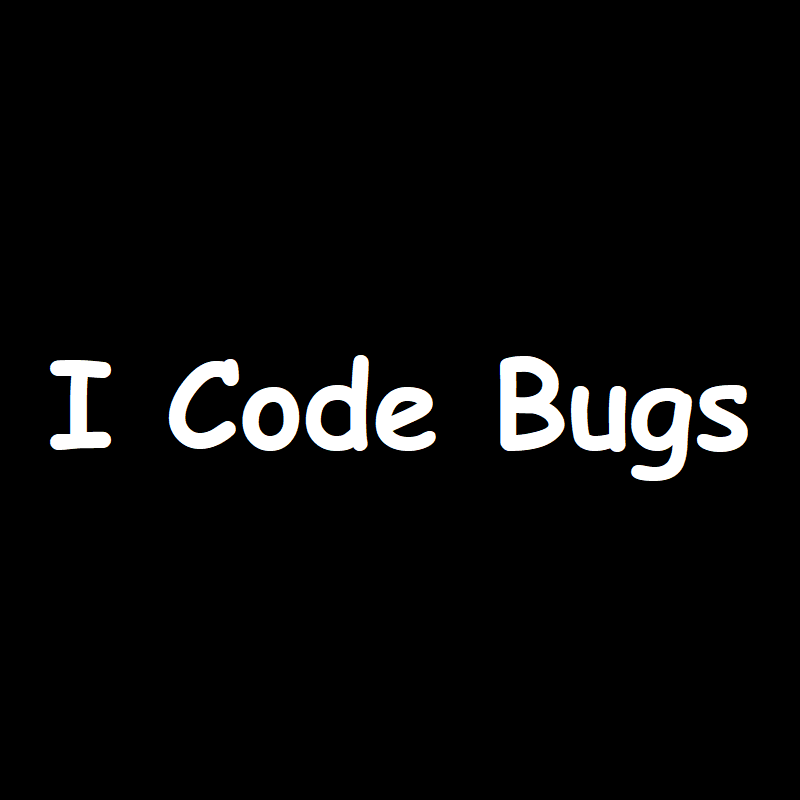# [LeetCode] Shortest Palindrome 最短回文串

+关注继续查看

Given a string S, you are allowed to convert it to a palindrome by adding characters in front of it. Find and return the shortest palindrome you can find by performing this transformation.

For example:

Given "aacecaaa", return "aaacecaaa".

Given "abcd", return "dcbabcd".

Credits:
Special thanks to @ifanchu for adding this problem and creating all test cases. Thanks to @Freezen for additional test cases.

C++ 解法一：

class Solution {
public:
string shortestPalindrome(string s) {
string r = s;
reverse(r.begin(), r.end());
string t = s + "#" + r;
vector<int> next(t.size(), 0);
for (int i = 1; i < t.size(); ++i) {
int j = next[i - 1];
while (j > 0 && t[i] != t[j]) j = next[j - 1];
next[i] = (j += t[i] == t[j]);
}
return r.substr(0, s.size() - next.back()) + s;
}
};

Java 解法一：

public class Solution {
public String shortestPalindrome(String s) {
String r = new StringBuilder(s).reverse().toString();
String t = s + "#" + r;
int[] next = new int[t.length()];
for (int i = 1; i < t.length(); ++i) {
int j = next[i - 1];
while (j > 0 && t.charAt(i) != t.charAt(j)) j = next[j - 1];
j += (t.charAt(i) == t.charAt(j)) ? 1 : 0;
next[i] = j;
}
return r.substring(0, s.length() - next[t.length() - 1]) + s;
}
}

C++ 解法二：

class Solution {
public:
string shortestPalindrome(string s) {
string t = s;
reverse(t.begin(), t.end());
int n = s.size(), i = 0;
for (i = n; i >= 0; --i) {
if (s.substr(0, i) == t.substr(n - i)) {
break;
}
}
return t.substr(0, n - i) + s;
}
};

Java 解法三：

public class Solution {
public String shortestPalindrome(String s) {
int i = 0, end = s.length() - 1, j = end;
char arr = s.toCharArray();
while (i < j) {
if (arr[i] == arr[j]) {
++i; --j;
} else {
i = 0; --end; j = end;
}
}
return new StringBuilder(s.substring(end + 1)).reverse().toString() + s;
}
}LeetCode 234. 回文链表 Palindrome Linked List
LeetCode 234. 回文链表 Palindrome Linked List
0 0LeetCode 409. Longest Palindrome

0 0LeetCode 336. Palindrome Pairs

0 0LeetCode 214. Shortest Palindrome

0 0LeetCode 125. Valid Palindrome

0 0【LeetCode】Palindrome Pairs（336）
Given a list of unique words. Find all pairs of distinct indices (i, j) in the given list, so that the concatenation of the two words, i.e. words[i] + words[j] is a 　　palindrome.
0 0LeetCode之Palindrome Number（回文数）
LeetCode之Palindrome Number（回文数）
0 0​请判断一个链表是否为回文链表。 Given a singly linked list, determine if it is a palindrome. 示例 1: 输入: 1->2 输出: false 示例 2: 输入: 1->2->2->1 输出: true 进阶：你能否用 O(n) 时间复杂度和 O(1) 空间复杂度解决此题？ Follow up:Could you do it in O(n) time and O(1) space? 解题思路： 首先是寻找链表中间节点，这个可以用快慢指针来解决，快指针速度为2，慢指针速度为1，快指针遍历完链表时，慢指针刚好走到中间节点（相对）。
360 0[LeetCode] Palindrome Permutation 回文全排列
931 0[LeetCode] Longest Palindrome 最长回文串
616 0